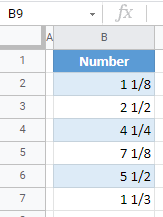# How to Convert Decimal to Fraction in Excel & Google Sheets

This tutorial demonstrates how to convert a decimal value to a fraction in Excel and Google Sheets.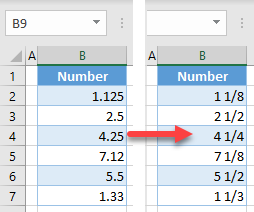## Convert Decimal to Fraction

In Excel, you can convert decimal numbers to fractions, by changing the format of the numbers. Say you have a list of decimal numbers in Column B.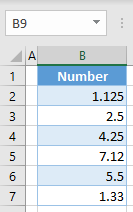1. To convert the values to fractions, first select the range of cells you want to convert (here, B2:B7).
2. In the Ribbon, go to the Home tab.
3. Click on the Number format drop-down.
4. Choose Fraction.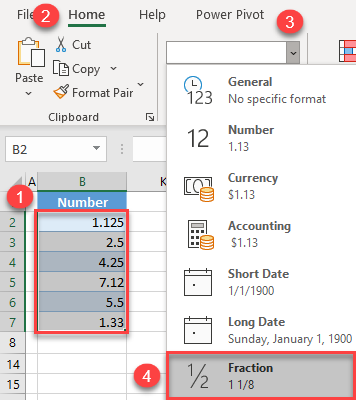As a result, all decimal numbers from the range are now formatted as fractions.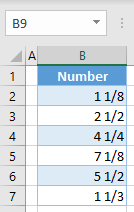As you can see in the picture above, all fractions have a one-digit denominator. Therefore, some values are rounded to the closest fraction with a one-digit denominator. In this example, the value in cell B5 (7.12) is converted to 7 1/8. If you want a more precise fraction, change the type, and show fractions with multiple-digit denominators.

1. Select the data range with fractions (B2:B7), and in the Ribbon, go to the Home tab, and click on the Number Format icon (in the bottom right corner of the Number group).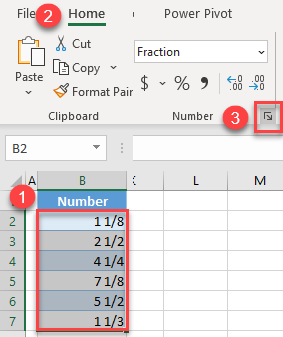1. In the Format Cells window, the Fraction category is already selected. For the Type, select Up to two digits (21/25) and click OK.
Note that there are other available fraction types (halves, quarters, eights, etc.), as you can see in the picture below.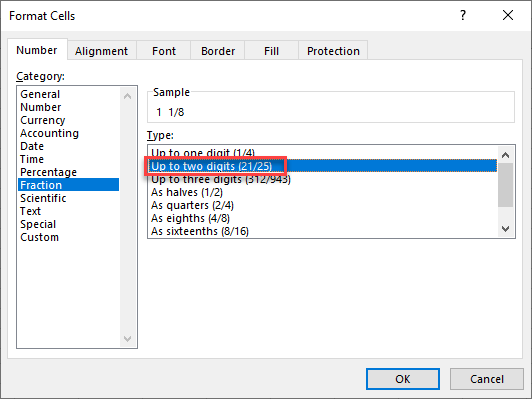Now, all fractions in the range have a two-digit denominator. This means that, if a fraction can’t be displayed exactly with a one-digit denominator, it is displayed with two digits.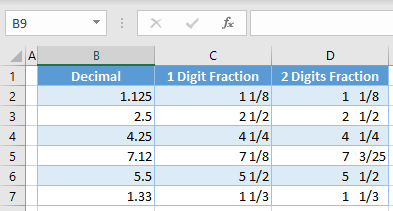Most of the fractions in this example can be reduced to a one-digit denominator, so those look the same regardless of fraction format type. However, the value in cell B5 (7.12) can’t be precisely presented with a one-digit denominator; it needs two digits: 7 3/25.

Note: When entering fractions between 0 and 1 (for example, 1/4 or 3/8), they can be incorrectly formatted as dates. To prevent this, stop Excel from auto-formatting dates.

## Convert Decimal to Fraction in Google Sheets

You can also convert decimals to fractions in Google Sheets.

1. After you select the data range you want to convert (B2:B7), in the Menu, go to Format > Number > More Formats > Custom number format.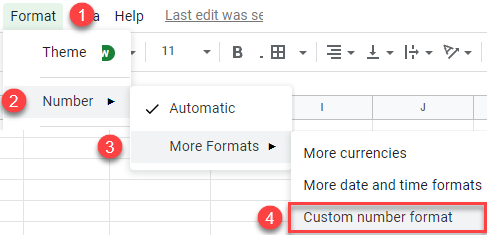1. In the Custom number formats menu, choose # ?/? and click Apply.
If you want two-digit denominators, select # ??/??.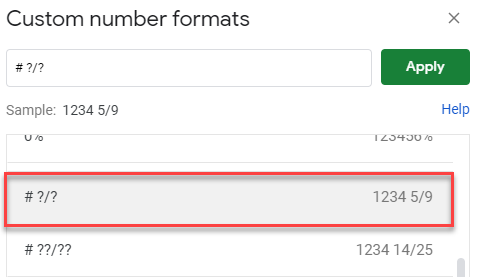As a result, all decimals in the range are converted to fractions.